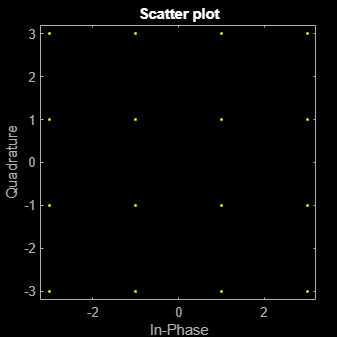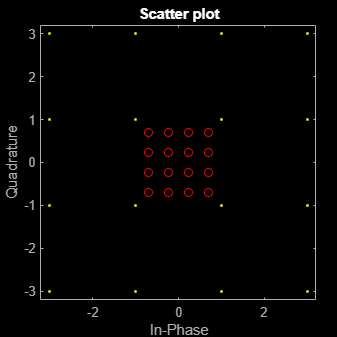# modnorm

Scaling factor for normalizing modulation output

## Syntax

``normfactor = modnorm(refconst,type,power)``

## Description

example

````normfactor = modnorm(refconst,type,power)` returns a scale factor for normalizing a PAM or QAM modulator output using the specified reference constellation, normalization type, and output power.```

## Examples

collapse all

Generate a 16-QAM reference constellation.

`refconst = qammod(0:15,16);`

Generate random symbols and apply 16-QAM modulation.

```x = randi([0 15],1000,1); y = qammod(x,16);```

Plot the constellation.

`h = scatterplot(y);`Compute the normalization factor so that the output signal has a peak power of 1 W.

```nf = modnorm(refconst,'peakpow',1); z = nf*y;```

Confirm that no element of the normalized signal has a power greater than 1 W.

`max(z.*conj(z))`
```ans = 1.0000 ```

Add the normalized constellation to the scatter plot.

```hold on scatterplot(z,1,0,'ro',h) hold off```## Input Arguments

collapse all

Reference constellation, specified as a vector of complex elements that comprise the reference constellation points.

Example: `qammod(0:15,16)`

Data Types: `double`
Complex Number Support: Yes

Normalization type, specified as either `'avpow'` or `'peakpow'`.

• If `type` is `'avpow'`, the normalization factor is calculated based on average power.

• If `type` is `'peakpow'`, the normalization factor is calculated based on peak power.

Data Types: `char`

Target power, specified as a real scalar. The target power is the intended power of the modulated signal multiplied by `normfactor`.

Data Types: `double`

## Output Arguments

collapse all

Normalization factor, returned as a real scalar. When a modulated signal is multiplied by the normalization factor, its average or peak power matches the target power. The function assumes that the signal you want to normalize has a minimum distance of 2.

## Version History

Introduced before R2006a